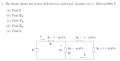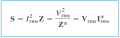# AC Circuits: Finding currents, impedances and voltages from power delivered to loads

#### YellowBelliedMarmot

Joined Jun 22, 2016
4
Problem:Relevant Formulas:

S = VI*
V = IZ
S = V^2 / Z

Attempt at Solution:
I know from my many attempts that my problem is not knowing when to use peak and rms values for current and voltage. I'll show the steps that I follow to get to each solution on the left, and then an explanation of my thought process on the right.

(a) This is quite easy; all I have to do is add up the loads and implement S = VI*

S = 7000 - j1000 Total load

S = (Vrms)(I*rms) Solve for I...
I*rms = (S) / (Vrms)
(I*) / sqrt(2) = (7000 - j1000) / (300 / sqrt(2)) Substitute in values...
I* / sqrt(2) = 33 - j4.714
I = 47.14 A at an angle of 8.13 degrees

I have confirmed that this value is correct.

(b) This is where things start to confuse me. I use the formula S = (I^2)(Z) to find the impedance of Z1

S = (Irms^2)(Z) Solve for Z...
Z = (S) / (Irms^2) Substitute in values....
Z = (5000 - j1000) / ((47.14 / sqrt(2))^2) Calculator....
Z = 4.5 - j2.7 Rectangular Form
Z = 5.25 ohms at and angle of -30.96 degrees Polar Form

I don't know if this is correct; however, I believe I may have made a mistake by using the rms value for the current (I).

(c) In order to find V2, I'll use KVL to get it. I'll find the voltage across the load S1 and subtract it from the source voltage.

S1 = (V1rms) (I*rms)
S1 = (1/2) (V1) (I*) Solve for V1...
V1 = ((2)*(S1)) / (I*) Substitute values in....
V1 = ((2)*(5000-i*3000)) / (47.14) Calculator...
V1 = 247.4 V at an angle of -30.96 degrees

Then I subtract the rms value of this voltage from the rms value of the source voltage to get V2...

V2 = Vrms - V1rms Substitute values in....
V2 = (300) / sqrt(2) - 247.4 / sqrt(2) Calculator....
V2 = 37.19 V at an angle of -30.96 degrees

I'm almost 100% sure that this value in wrong. I can't prove it, but I have a gut feeling it is.

(d) In order to find the impedance Z2, I will use the formula S = V^2 / Z

S2 = (Vrms)^2 / Z2
Z2 = (Vrms)^2 / S2 Solve for Z2
Z2 = (37.19 / sqrt(2))^2 / (-j8000) Sub in values and calculate...
Z2 = j0.08644 ohms
Z2 = 86.4 milli ohms at an angle of 90 degrees

I know for a fact that this is incorrect. The answer should be 900 milli ohms at an angle of -90 degrees. On the bright side, my degrees is similar, just an opposite sign. If I had to guess, I may have dropped a conjugate I* somewhere. However, the amount of milli ohms is way off. If I had to guess what I did wrong, I think I may have accidentally used an rms value when I should've used a peak value perhaps?

(e) I'm not going to post my work for e as my work up until this point would simply result in another wrong answer. I think it'd be best if I start from the beginning and figure what I did wrong first and then continue on.

#### WBahn

Joined Mar 31, 2012
28,488
It will help you (and us) a lot if you adopt a notation that makes it clear when you are talking about voltage and current amplitudes and when you are talking about effective voltages and currents.

The most common such convention is to use Ip and Vp for amplitudes and Irms and Vrms for effective values.

•YellowBelliedMarmot

#### YellowBelliedMarmot

Joined Jun 22, 2016
4
It will help you (and us) a lot if you adopt a notation that makes it clear when you are talking about voltage and current amplitudes and when you are talking about effective voltages and currents.

The most common such convention is to use Ip and Vp for amplitudes and Irms and Vrms for effective values.
That's my main problem; I don't exactly know when to use the I peak or V peak as opposed to rms (i.e. effective). But I think what you're referring to is part (c); I can see what you mean that things might not be clear since I dropped the rms suddenly by converting Vrms ---> Vp/sqrt(2)). My bad! I'll put that part below again to clarify if that would help. Let me know if there's anything else that I should clarify!

(c) In order to find V2, I'll use KVL to get it. I'll find the voltage across the load S1 and subtract it from the source voltage.

S1 = (V1rms) (I*rms) V1rms = V1p / sqrt(2) & I*rms = I*p / sqrt(2)
S1 = (V1rms) (I*rms) Solve for V1... (I multiplied both sides by sqrt(2) to get V1p alone)
V1p / sqrt(2) = ((2)*(S1)) / (Ip* / sqrt(2)) Substitute values in....
V1p = sqrt(2) * ((5000-i*3000)) / (47.14 / sqrt(2)) Calculator...
V1p= 247.4 V at an angle of -30.96 degrees

Then I subtract the rms value of this voltage from the rms value of the source voltage to get V2...

V2p = Vrms - V1rms Substitute values in....
V2p = (300) / sqrt(2) - 247.4 / sqrt(2) Calculator....
V2p = 37.19 V at an angle of -30.96 degrees

#### WBahn

Joined Mar 31, 2012
28,488
To know when to use which, you need to understand what each of them means. In AC analysis (in which we are dealing with pure sinusoidal waveforms), the amplitude (peak value) is the value that multiplies the sinusoid to yield the actual time-varying signal. The rms value is an effective value used for power computations because it is the value that a DC signal would have to have in order to dump the same amount of power, on average, in a resistor that the actual sinusoidal waveform would.

So in part (a), is your answer for "I" peak or rms? Label it properly and it will be much easier to use the right value in part (b) (note that I am not saying one way or the other whether you used the right value, but the fact that you are unsure whether you did or not means that it's a good time to take a pause and make sure that you DO know which value is the right one to use.

•YellowBelliedMarmot

#### RBR1317

Joined Nov 13, 2010
706
That's my main problem; I don't exactly know when to use the I peak or V peak as opposed to rms (i.e. effective).
Consider whether the complex power 'S' values are given in terms of rms or peak values. Also consider whether the 'V' & 'I' terms are just rms values or are phasors with an rms magnitude. It might be helpful to check your relevant formulas against the attached extract from "Fundamentals of Electric Circuits" by Alexander & Sadiku.

#### Attachments

• 259.4 KB Views: 2
•YellowBelliedMarmot

#### WBahn

Joined Mar 31, 2012
28,488
Consider whether the complex power 'S' values are given in terms of rms or peak values. Also consider whether the 'V' & 'I' terms are just rms values or are phasors with an rms magnitude. It might be helpful to check your relevant formulas against the attached extract from "Fundamentals of Electric Circuits" by Alexander & Sadiku.
What does it mean for power to be in rms or peak values? What would "watts-rms" mean?

•YellowBelliedMarmot

#### YellowBelliedMarmot

Joined Jun 22, 2016
4
Consider whether the complex power 'S' values are given in terms of rms or peak values. Also consider whether the 'V' & 'I' terms are just rms values or are phasors with an rms magnitude. It might be helpful to check your relevant formulas against the attached extract from "Fundamentals of Electric Circuits" by Alexander & Sadiku.Ok, this is going to help me narrow down my question.

First, when it says Z*, what does it want? Does it want the conjugate of the impedance in rectangular form?

Second, when it says I*rms, what exactly is that? I've always been confused about that because how can you give a conjugate of an rms value? Isn't it only a single number: Ip / sqrt(2) ?

Thanks for the replies by the way! I'm in an 8 week online circuits class, and I'm having a hard time finding good resources, especially for complex power (perhaps that's why they call it complex? ;D).

#### YellowBelliedMarmot

Joined Jun 22, 2016
4
To know when to use which, you need to understand what each of them means. In AC analysis (in which we are dealing with pure sinusoidal waveforms), the amplitude (peak value) is the value that multiplies the sinusoid to yield the actual time-varying signal. The rms value is an effective value used for power computations because it is the value that a DC signal would have to have in order to dump the same amount of power, on average, in a resistor that the actual sinusoidal waveform would.

So in part (a), is your answer for "I" peak or rms? Label it properly and it will be much easier to use the right value in part (b) (note that I am not saying one way or the other whether you used the right value, but the fact that you are unsure whether you did or not means that it's a good time to take a pause and make sure that you DO know which value is the right one to use.
Yes, in part (a) the value that I found was the peak current.

S = (Vrms)(I*rms)_________________________________Solve for I*rms...
I*rms = (S) / (Vrms)
(Ip*) / sqrt(2) = (7000-j1000)/(300/sqrt(2))______________I*rms = Ip* / sqrt(2) & Vrms = 300 / sqrt(2)
Ip* / sqrt(2) = 33 - j4.714___________________________Solve for Ip* and take the conjugate...
Ip = 47.14 A at an angle of 8.13 degrees

#### WBahn

Joined Mar 31, 2012
28,488
View attachment 108164
Ok, this is going to help me narrow down my question.

First, when it says Z*, what does it want? Does it want the conjugate of the impedance in rectangular form?
The superscript * is the operator for complex conjugation (it matters not what form the complex number is written in -- it's the complex number that is being conjugated, not its representation - the mechanics of performing the conjugation depends on the representation used, but they are all equivalent).

Second, when it says I*rms, what exactly is that? I've always been confused about that because how can you give a conjugate of an rms value? Isn't it only a single number: Ip / sqrt(2) ?
The voltages and currents are phasors which have magnitude and phase (or, equivalently, a real and an imaginary part). The values used can be either amplitudes or effective values. The rms subscript means that you are using effective values.

Thanks for the replies by the way! I'm in an 8 week online circuits class, and I'm having a hard time finding good resources, especially for complex power (perhaps that's why they call it complex? ;D).
This might help: